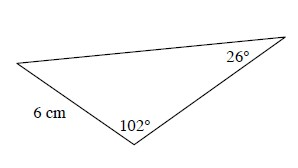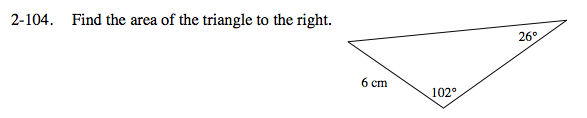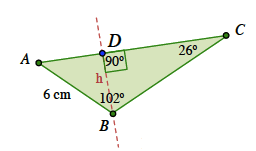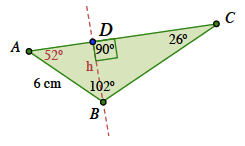### Home > PC > Chapter 2 > Lesson 2.3.3 > Problem2-104

2-104.

Find the area of the triangle below. Homework Help ✎Draw a perpendicular line from angle B to side AC as shown in the diagram at right to get a height of the triangle.

Find angle A by subtracting (102 + 26) from 180.

Find h.

Use the Law of Sines to get the base AC.

$\text{Area} =\frac{1}{2}\text{base }\cdot \text{ height}$

$\text{Area }=\frac{1}{2}(AC)(h)$

Substitute the appropriate numbers to find the answer.$\text{Sin}52\degree=\frac{h}{6}\ \ \ \ \ h=6\text{ Sin}52\degree$

$\frac{6}{\text{Sin}26\degree}=\frac{AC}{\text{sin}102\degree}$

$AC=(\text{sin}102\degree)\frac{6}{\text{Sin}26\degree}$